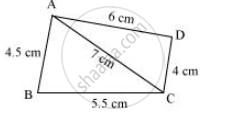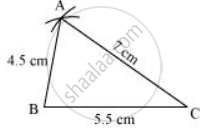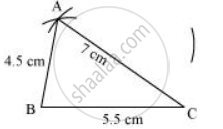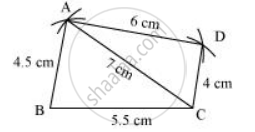Share

# Construct the Following Quadrilaterals. Quadrilateral Abcd Ab = 4.5 Cm, Bc = 5.5 Cm - Mathematics

Course
ConceptConstructing a Quadrilateral When the Lengths of Four Sides and a Diagonal Are Given

AB = 4.5 cm

BC = 5.5 cm

CD = 4 cm

AC = 7 cm

#### Solution

Firstly, a rough sketch of this quadrilateral can be drawn as follows.1) ΔABC can be constructed by using the given measurements as follows.2) Vertex D is 6 cm away from vertex A. Therefore, while taking A as centre, draw an arc of radius 6 cm.3) Taking C as centre, draw an arc of radius 4 cm, cutting the previous arc at point D. Join D to A and C.